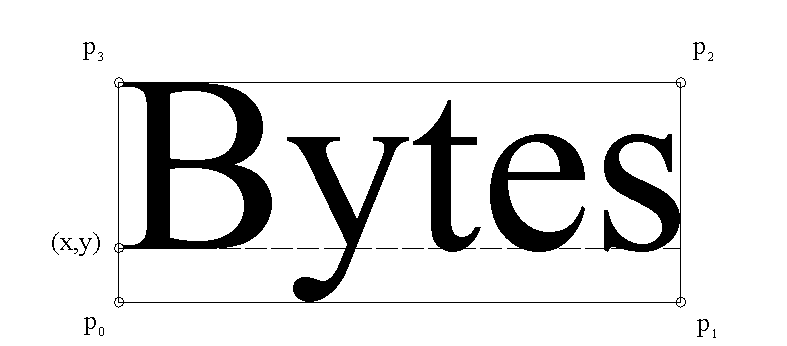Draw Text

Purpose

The Draw Text function displays a unicode string in the current font.

Syntax

 Opcode format Opcode [ASCII] (Hex) Operand Format Comments Single-byte, binary operand [ctrl-X] (18) []+ Basic unicode text, relative coordinates. Single-byte, binary operand [x] (78) [][[]]*[]\ [[]]*[]+ Advanced unicode text, relative coordinates.

f The rotation angle (in 360/65,536ths of a degree) of the text.

H The height of the text in logical coordinates.

x,y The insertion point of the text in logical coordinates.

count The number of unicode characters in the text string. A value of zero indicates that an extended count will follow.

Ecount When count is zero, a two-byte extended count follows. This allows for text strings of length 256 through 65791 which are encoded as an integer in the range 0 to 65,535.

Ci The ith unicode character in the text string.

ws The width scale (scaled by 1024; in other words, 1024=1.0).

ics The intercharacter spacing (scaled by 1024; in other words, 1024=1.0).

f Text flags reserved for future use.

q The oblique angle (in 360/65,536ths of a degree) of the text.

os-count One plus the number of overscore position indices for the text. (A value of one indicates no overscores). A value of zero indicates that an extended count will follow.

os-Ecount When os-count is zero, a two-byte extended count follows. This allows for an overscore index count of 256 through 65791 which are encoded as an integer in the range 0 to 65,535.

os-pos-i The ith overscore position index. A value of zero indicates that an extended count will follow.

os-Epos-i When os-pos-i is zero, a two-byte extended count follows. This allows for positions of 256 through 65791 which are encoded as an integer in the range 0 to 65,535.

us-count One plus the number of undererscore position indices for the text. (A value of one indicates no overscores). A value of zero indicates that an extended count will follow.

us-Ecount When us-count is zero, a two-byte extended count follows. This allows for underscore index count ofof 256 through 65791 which are encoded as an integer in the range 0 to 65,535.

us-pos-i The ith underscore position index. A value of zero indicates that an extended count will follow.

us-Epos-i When us-pos-i is zero, a two-byte extended count follows. This allows for positions 256 through 65791 which are encoded as an integer in the range 0 to 65,535.

D0, D1, ..., D8 Specify the text bounding box in logical coordinates relative to the insertion point. For more information, see "Notes".

NotesFigure 1. Text string bounding box

The bounding box for the string "Bytes" is shown in figure 1. The corners of the bounding box are specified relative to the insertion point (x,y) by the following:

D0 = p3,x - p0,x

D1 = p3,y - p0,y

D2 = p2,x - p1,x

D3 = p2,y - p1,y

D4 = p0,x - x

D5 =p1,y - p0,y

D6 = p0,y - y

D7 = p1,x - p0,x

Implementation in WHIP! Versions

 Toolkit Function TBD Partial Full Revised in Draw Text - - 2.0 -